The method described above is readily applied to observed data, and, as a second example consider the Old Faithful data set again.

The curve shown in figure 4 is the density, estimated using the above methods and a non-informative prior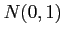, showing the two main modes.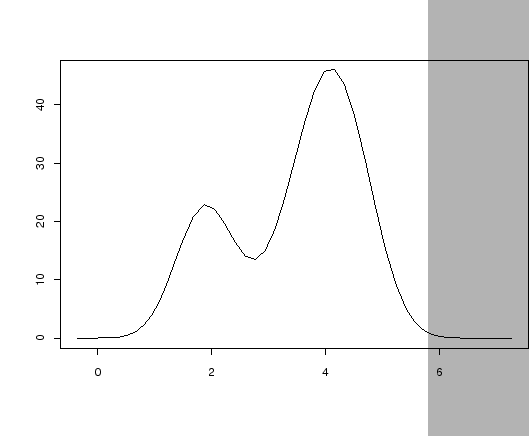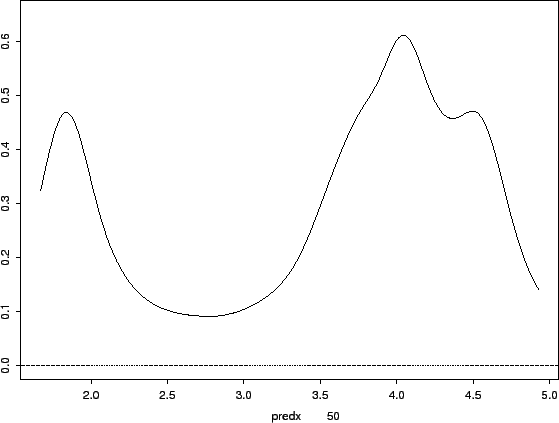As can be seen from the estimate, the density has two areas of high density and three of relatively low density. Intuitively a large bandwidth is required in an area of low density and a small bandwidth in an area of high density, smoothing out the tails and revealing more detail in the high density areas. This leads to the notion of an adaptive, two pass, estimate where the bandwidth is inversely proportional to the local density.

An estimate is required that works in a similar way. An initial KDE is used to modify the bandwidth used in the final estimate.

First a fixed bandwidth KDE is adopted as pilot estimate, so that, for such a pilot, on a sample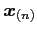(21)

where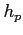is the pilot bandwidth. Following Abramson (1982) and taking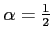ingives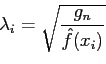(22)

as the local bandwidth. For univariate data with (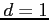) equation () gives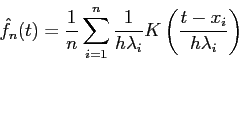(23)

where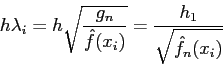(24)

The factor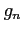is absorbed in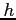as discussed in section. So it is seen that (23) is of the form(25)

This is similar to (2) and is still easily accommodated in the formulation based on (3).

Taking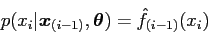(26)

a likelihood for the analysis can be constructed as before.

Figure 5 shows the density obtained from the eruption duration subset of the Old Faithful data using the adaptive KDE. The two main peaks are still there, however there is a third peak appearing at around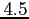minutes that is not obvious in the simple estimate. This is also seen in Figure 6 and, to a slightly reduced level, in the larger data set in Figure 74 (this data has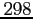items as opposed to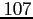).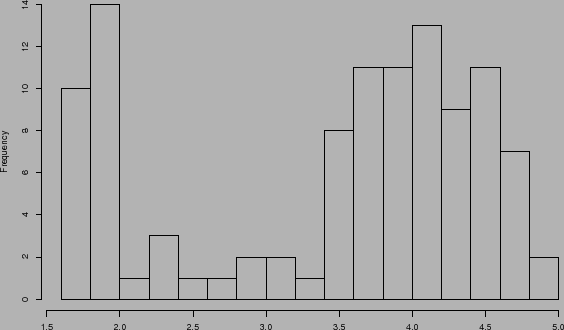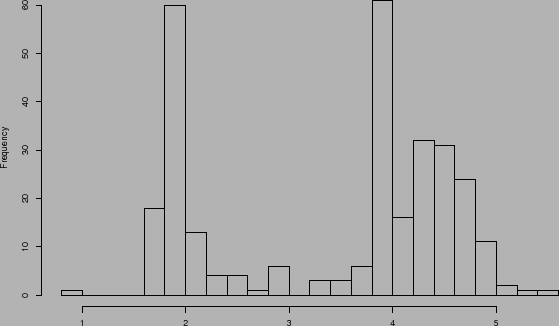danny 2009-07-23